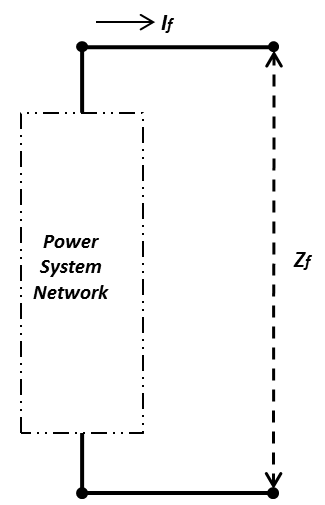# Impedance Method Short Circuit Calculation

Any power system network (Figure 1) can be represented by a simplistic Thevenin's equivalent circuit (Figure 2). Using the Thevenin's equivalent circuit of the network, it will be very easy to calculate the fault current at any point of the the power system.Figure 1 Power System NetworkFigure 2 Thevenins Equivalent Circuit

where:''From Figure 2, the fault current will beMost faults are considered to be bolted faults thus, which will simplify the equation to